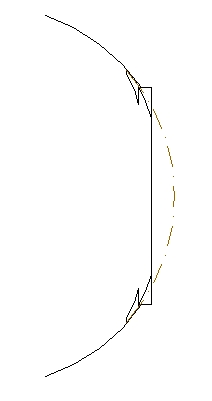FE stress analysis for a first outline of the vacuum vessel of UST_1 Abstract :  The calculation of the stress in a porcelain vacuum vessel for the UST torus is described. The only load considered in this calculation is the atmospheric pressure. Magnetic forces are very low if only a basic set of power supplies is used. The objective is to continue experimenting with the code_ASTER and to obtain an approximate value of the stress in the structure..      Modelling         The major radius of the torus is 0.1125 m . The minor radius of the external surface of the torus is 0.04775 m and the thickness of the wall is 10mm. The reason of the relatively thick wall is the specific process of moulding that is foreseen and to allow attaching the external flanges to the non-protuberant ports of the torus. A figure representing the present idea about this type of ports is shown in Graph 2. The model for the finite elements code, ASTER, has been simplified. Instead of 5 ports like the one in Graph 2 only one was modelled. The structure of the port was reduced to a square hole. See the design in Graph 1. The port is at the bottom left of the figure.    The atmospheric pressure on the port section is applied on the borders of the rectangular port. A pressure of 1 bar = 100000 N/m2 is applied on the rest of the external surface of the torus and perpendicular to each element of the surface.    The electro-magnetic forces are small, about 4N/linear-cm of coil if the current in the HF coil is 710A. See calculations in . Therefore these forces are not applied in the calculation.        Non-protuberant ports      The general structure of the torus is thought to be similar to CTH, m=5 , l=2,  , but only 5 equatorial ports are planned.   The ports are thought to be flat to allow changing the shape and distribution of the windings on the surface of the torus. In Graph 3 a profile of the port is displayed.    Dashed lines in Graph 3 represent the mathematical section of the torus and continuous lines the contour of the port. Only two small parts at the top and bottom of the port protrude a few millimetres. The necessity of nuts to fix the port is the reason of this extensions. This configuration forces the use of two stuck nuts. The porcelain torus should be moulded by mean of a tapping process. As a consequence the thickness of the wall remains constant even if 4 slits like the ones in Graph 2 are planned for 4 nuts.    In this design part of the ports can be covered by coils in order to admit future unknown configurations. The number of periods could even be changed to l=3, l=4 or other. Moreover the TF coils for a classical stellarator, style WEGA for example, will fit over the ports.       Solution using ASTER      The general process of meshing with GMSH and solving with ASTER is described in         The present model uses "3D  modellisation" and the constraints are supposed to be on a ring on the internal hole of the torus.       The properties of the material are: Young’s modulus 137GPa  ;  Poisson’s ratio 0.19  corresponding  to an average Zircon porcelain.    All this values were defined using EFICAS, a tool to easily define the FEA model in ASTER.           The distribution of Von Mises stress around the port is represented in Graph 4. The maximum value is 1.36 x 106Pa and the minumum 1.1x106Pa .  Maximum values are around the port.    The stress, as was expected, is very small and far from the tensile strenght of an average zircon porcelain, about 700-1000 Kg/cm2 .         Further developments    If more power supplies were available the magnetic forces should be introduced in the model and the maximum current would be calculated.     References  "Forces on HF coil of the first outline of the UST_I" . See "All past research" in this web.  “Design and construction progress of the compact toroidal hybrid" Hartwell, G.J., Knowlton, S.F. Watts, C.Hanson, J.D., Brown, T.  "FE stress analysis of the case for the TF coils in HT-7U using Code_ASTER and GMSH" . See "All past research" in this web. Graph 4 . Von Mises stress around the port           Graph 1  .  Deformation of the TF coil under out-of-plane loads. Units in  meters. Calculation using ASTER-GMSH             Graph 2  .  Present ideas about a non-protuberant port.Graph 3  .  Distribution of Von Mises stress. Units in  Pa.  Calculation using ASTER-GMSH         Graph 4 . Von Mises stress around the port Last Update 22-07-2005